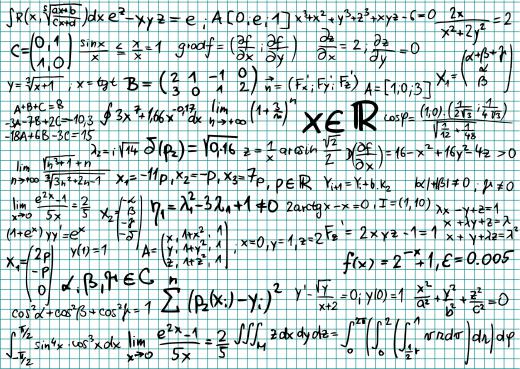Science
Fact Checked

# What Are Confidence Intervals?

C.H. Seman
C.H. Seman

In statistics, confidence intervals are used as interval estimates for population parameters. They frequently are used in science and engineering for hypothesis testing, statistical process control and data analysis. Although it is possible to calculate confidence intervals by hand, it typically is easier and much faster to use specialized statistics programs or advanced graphing calculators.

If a probability statement of the form P(L≤θ≤U) = 1 - α can be written such that L and U are exclusively functions of the sample data and θ is a parameter, then the interval between L and U is a confidence interval. This definition can be stated in a more intuitive and practical manner by saying that a statement that the parameter θ lies in the confidence interval will be true 100(1 - α)% of the times the statement is made. The term (1 - α) is known as the confidence coefficient.

For the case of a normally distributed population with known mean μ and known variance σ2, the 100(1 - α) confidence interval around the mean can be calculated by the equation x - zα/2σ/√n ≤ μ ≤ x + zα/2σ/√n, in which zα/2 is the upper 100α/2 percentage point of the standard normal distribution curve. This is a simple case, because the true mean and variance of the entire population usually are not known.Confidence intervals most often are used to determine how well a certain parameter fits within a given data set.

Confidence intervals most often are used to determine how well a certain parameter fits within a given data set. For example, if the confidence interval for a given data set spans from 45 to 55 with a confidence coefficient of 0.95, one could argue that any data point that falls within this region belongs in the population with 95 percent confidence. Increasing the confidence coefficient tightens the interval, meaning a smaller range of variables can be explained with greater confidence. Decreasing the confidence coefficient broadens the interval but decreases the confidence.

For some applications, such as normally distributed populations with known means and variances, the equations used to calculate confidence intervals are readily available. Statistics tables can be used to find values for zα/2. Other applications, such as data analysis in engineering, require more sophisticated methods of calculation. It usually is more practical to use a statistics program to determine confidence intervals for these cases. Statistics programs can be especially useful when data sets are extremely large and results must be presented graphically.

## You might also Like